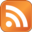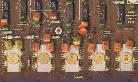# Robotrontechnik-ForumWillkommen Gast!Robotrontechnik-Forum » Diskussionen über das Forum und robotrontechnik.de » Bilder auf der Website » Themenansicht

000
23.02.2014, 15:08 Uhr
dk_spb

Wie kann ich Bilder von der robotrontechnik.de zu bekommen?
beispielsweise http://www.robotrontechnik.de/bilder/Komponenten/Platinen/K1520/012-7060_K7022_ABD_i.jpg

How I can get the normal size pictures from robotrontechnik.de?
For example http://www.robotrontechnik.de/bilder/Komponenten/Platinen/K1520/012-7060_K7022_ABD_i.jpg
 Zitatantwort || Editieren || Löschen
001
24.02.2014, 08:17 Uhr
holmThis simple doesn't work because of software lmitations on the Server... Rüdiger sayd...

Regards,

Holm
--
float R,y=1.5,x,r,A,P,B;int u,h=80,n=80,s;main(c,v)int c;char **v;
{s=(c>1?(h=atoi(v)):h)*h/2;for(R=6./h;s%h||(y-=R,x=-2),s;4<(P=B*B)+
(r=A*A)|++u==n&&putchar(*(((--s%h)?(u<n?--u%6:6):7)+"World! \n"))&&
(A=B=P=u=r=0,x+=R/2))A=B*2*A+y,B=P+x-r;}
 Zitatantwort || Editieren || Löschen
002
24.02.2014, 16:31 Uhr
dk_spb

server on PC1715 ?
May be does it possible make archive file with pictures and publish it on another site?
Doesn't possible to see anything on these small photos.
There are no any problem if I know that there are no any photos.
But, if I know that photos exist - it is not so good for me ;-)
 Zitatantwort || Editieren || Löschen
003
24.02.2014, 22:01 Uhr
holmHmm, I've diskussed exactly this problem some years ago here.
Maybe I should talk about this with the other guys here. I can host the pics easily
but I don't habe much time to take pictures and so on...

Lets seee..

Regards,

Holm
--
float R,y=1.5,x,r,A,P,B;int u,h=80,n=80,s;main(c,v)int c;char **v;
{s=(c>1?(h=atoi(v)):h)*h/2;for(R=6./h;s%h||(y-=R,x=-2),s;4<(P=B*B)+
(r=A*A)|++u==n&&putchar(*(((--s%h)?(u<n?--u%6:6):7)+"World! \n"))&&
(A=B=P=u=r=0,x+=R/2))A=B*2*A+y,B=P+x-r;}
 Zitatantwort || Editieren || Löschen
 Seiten: -1-     [ Diskussionen über das Forum und robotrontechnik.de ]

 Robotrontechnik-Forum- Technische Diskussionen- Veranstaltungen / Termine- Flohmarkt- Sonstiges- Diskussionen über das Forum und robotrontechnik.de Admin:   Schließen/Öffnen   Löschen   Verschieben   Festmachen/Lösen

Robotrontechnik-Forum